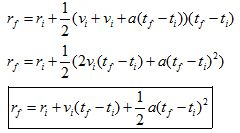$$\require{cancel}$$

# 02. Deriving the kinematic relationships

•• Contributed by Paul D'Alessandris
• Professor (Engineering Science and Physics) at Monroe Community College

Let’s construct the two independent kinematic relationships that you will use whenever the acceleration is constant. In a later chapter, we will return to the case in which the acceleration is not constant.

From the definition of acceleration:The above relationship is our first kinematic relationship. The acceleration in this relationship is really the average acceleration. However, since the acceleration is constant, the average acceleration is the same as the acceleration at any instant between the initial and final state.

From the definition of velocity:You must remember, however, that the velocity in this formula is really the average velocity of the object over the time interval selected. To keep you from having to remember this fact, we can rewrite the average velocity as the sum of the initial velocity and the final velocity divided by two:Substituting in the first kinematic relationship for the final velocity yields:The above relationship is our second kinematic relationship.

Although we could keep deriving new kinematic equations forever, it is impossible that any other derived equation could allow us to calculate some quantity that these equations do not allow us to calculate.